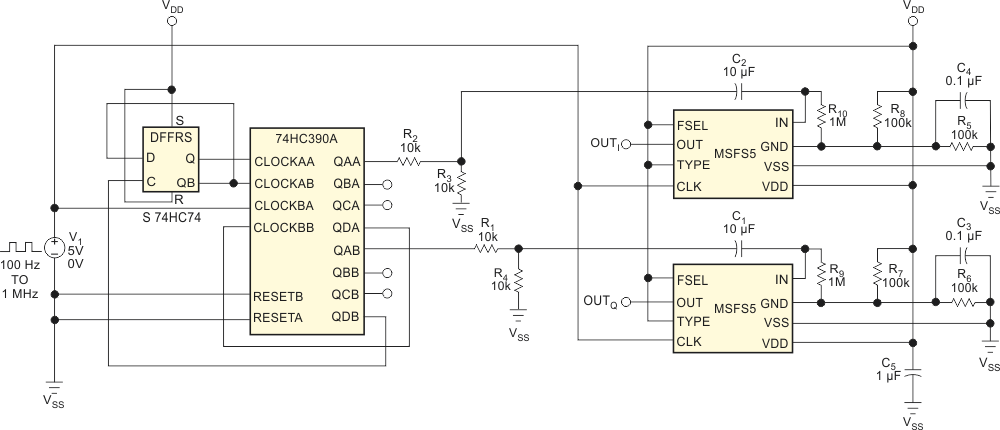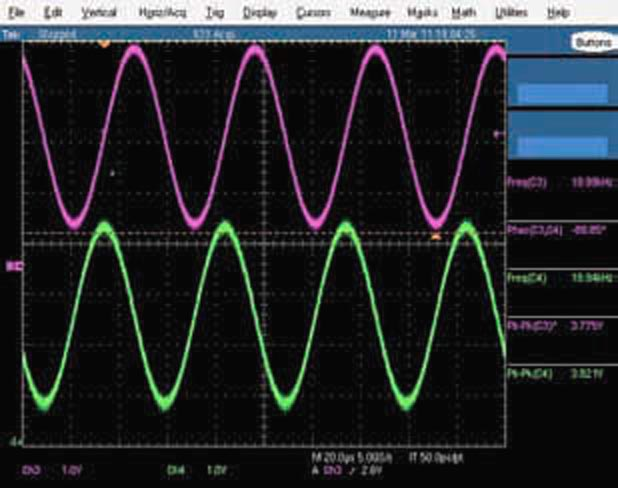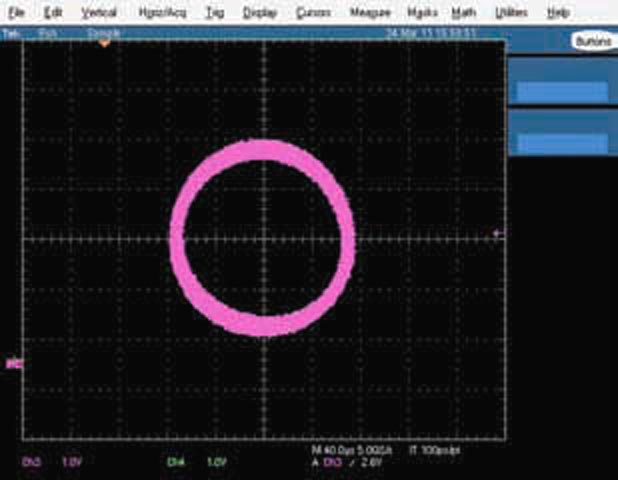# Generate swept sine/cosine waveforms with two filters

## Texas Instruments CD74HC390 SN74HC74

John R Ambrose

EDN

Demodulators, directional circuits, and other electronics applications often need two sine waves with a 90° difference in phase – a sine wave and its cosine wave. Engineers typically use analog filters to create the phase shift. This approach, however, offers a limited frequency range. Using the circuit in Figure 1, you can make a swept sine/cosine pair at frequencies of less than 1 Hz to 25 kHz.Figure 1. This circuit lets you make a swept sine/cosine pair at frequencies of less than 1 Hz to 25 kHz.

The Mixed Signal Integration MSFS5 selectable lowpass/bandpass switched-capacitor filter removes the harmonics from a square wave you apply to its inputs. The clock for the MSFS5 is 100 times the input square wave. The 74HC390 and 74HC74 form a divide-by-25 and a divide-by-two circuit. The Q outputs from the 74HC74 connect to the two divide-by-two circuits in the 74HC390A, which produces square waves that are 1/100 of the filter clock’s frequency and are 90° out of phase from each other. A square wave at CMOS levels would saturate the filter, so the circuit uses resistor dividers R1 through R4 to reduce the signal’s amplitude.Figure 2. The phase reading on the scope is –89.85°.

Figure 2 shows the output of the two filters at 20 kHz with a system clock of 2 MHz. Note that the phase reading on the scope is at –89.85°. When swept in frequency, the phase varies from –89° to –91°. Figure 3 shows a 20-kHz Lissajous pattern.Figure 3. When swept in frequency, the phase varies from –89° to –91°.

Measuring the circuit’s distortion using a spectrum analyzer and an Audio Precision audio analyzer shows a THD (total harmonic distortion) of –49 dB. Testing shows that the circuit has no discontinuity at the filter outputs with either FSK (frequency-shift keying) or FM (frequency modulation).

EDN